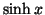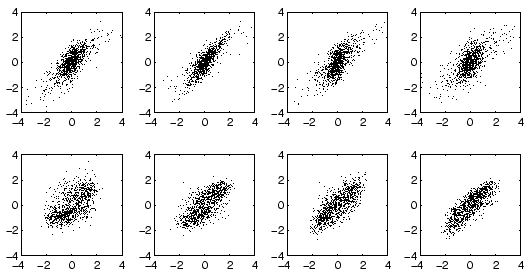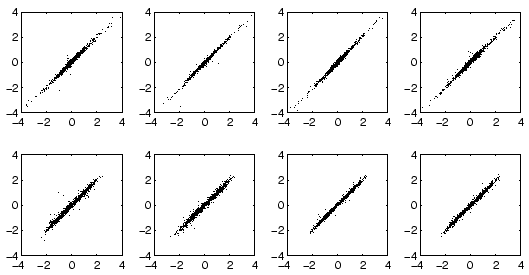Next: Process Data Up: Nonlinear Artificial Data Previous: Gaussian Sources.

### Non-Gaussian Sources.

The following experiments demonstrate the ability of the nonlinear independent factor analysis algorithm to find the underlying latent variables which have generated the observations.

In these experiments, a similar scheme was used to generate data as with the Gaussian sources before. Now a total of eight sources was used with four sub-Gaussian and four super-Gaussian sources. The generating MLP network was also larger, having 30 hidden neurons and 20 output neurons. The super-Gaussian sources were generated by taking a hyperbolic sine,, from a normally distributed random variable and then normalising the variance to unity. In generating sub-Gaussian sources, inverse hyperbolic sine,, was used instead of.

Several different numbers of hidden neurons were tested in order to optimise the structure of the network, but the number of sources was assumed to be known. This assumption is reasonable since it is possible to optimise the number of sources simply by minimising the cost function as the experiments with the Gaussian sources show. The network which minimised the cost function turned out to have 50 hidden neurons. The number of Gaussians in each of the mixtures modelling the distribution of each source was chosen to be three and no attempt was made to optimise this.The results are depicted in Figs. 11, 12 and 13. Each figure shows eight scatter plots, each of which corresponds to one of the eight sources. The original source which was used for generating the data is on the x-axis and the estimated source in on the y-axis of each plot. Each point corresponds to one data vector. The upper plots of each figure correspond to the super-Gaussian and the lower plots to the sub-Gaussian sources. Optimal result would be a straight line which would mean that the estimated values of the sources coincide with the true values.

Figure 11 shows the result of a linear FastICA algorithm . The linear ICA is able to retrieve the original sources with 0.7 dB signal to noise ratio. In practise a linear method could not deduce the number of sources and the result would be even worse. The poor signal to noise ratio shows that the data really lies in a nonlinear subspace.

Figure 12 depicts the results after 2000 iterations with nonlinear factor analysis followed by a rotation with a linear FastICA. Now the signal to noise ratio is 13.2 dB and the sources have clearly been retrieved. Figure 13 shows the final result after another 5500 iterations with nonlinear independent factor analysis algorithm. The signal to noise ratio has further improved to 17.3 dB.

It would also be possible to avoid using the nonlinear independent factor analysis algorithm by running first 7500 iterations with linear factor analysis algorithm and then applying the linear ICA. The disadvantage would be that the cost function would not take into account the non-Gaussianity. The signal to noise ratio after 7500 iterations with linear factor analysis algorithm followed by the linear ICA was 14.9 dB, which shows that taking the non-Gaussianity into account during estimation of the nonlinear mapping helps the nonlinear independent factor analysis algorithm to find better estimates for the sources.Next: Process Data Up: Nonlinear Artificial Data Previous: Gaussian Sources.
Harri Lappalainen
2000-03-03